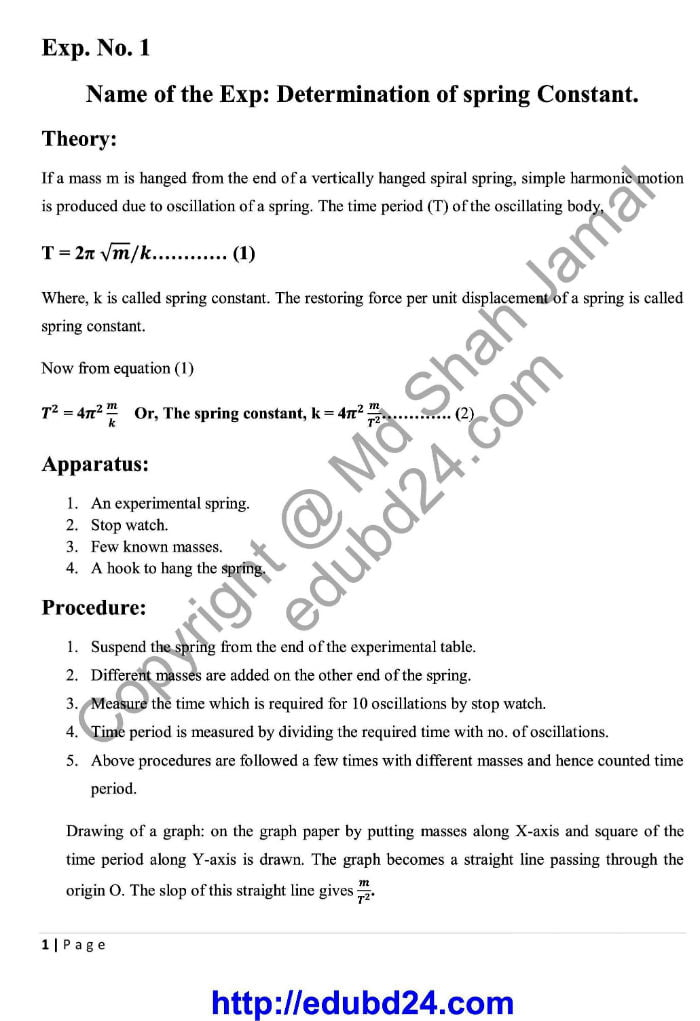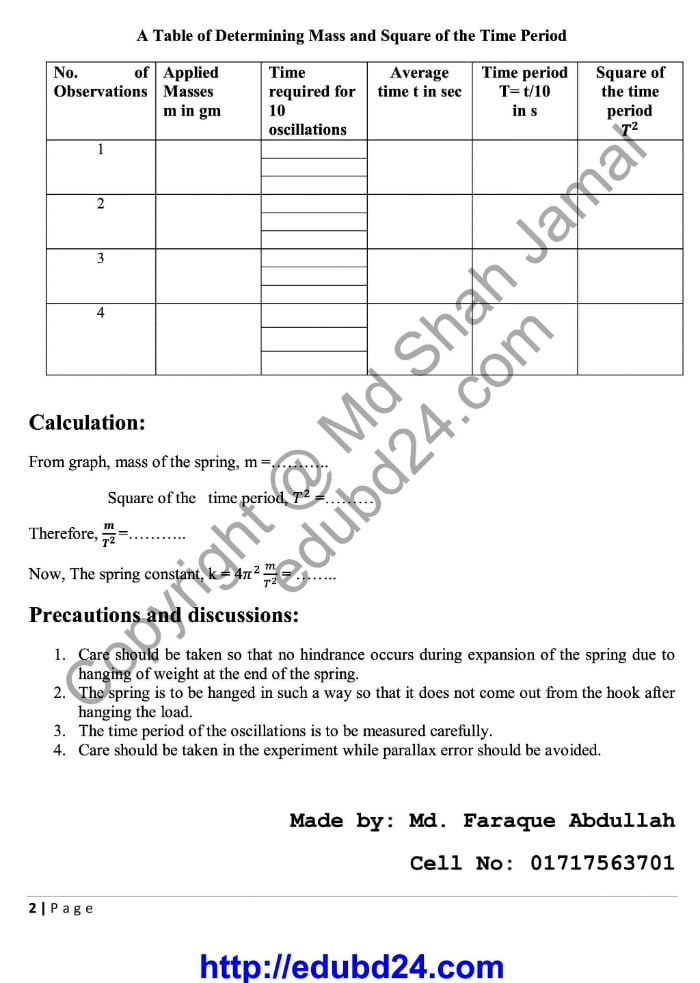# Determination of spring Constant

Determination of spring Constant (English Version ). Determination of the Spring Constant of a Spring. Any physicist knows that if an object applies a force to a spring, then the spring applies an equal and opposite force to the object. Hooke’s law gives the force a spring exerts on an object attached to it with the following equation: F = –kx

where the minus sign shows that this force is in the opposite direction of the force that’s stretching or compressing the spring. (k is called the spring constant, which measures how stiff and strong the spring is. x is the distance the spring is stretched or compressed away from its equilibrium or rest position.)

## Determination of spring Constant# (English Version)

##Click Here to Download the above File

Determination of spring Constant

Determination of spring Constant

Determination of spring Constant

Determination of spring Constant

Determination of spring Constant